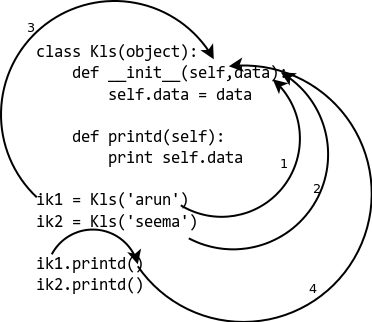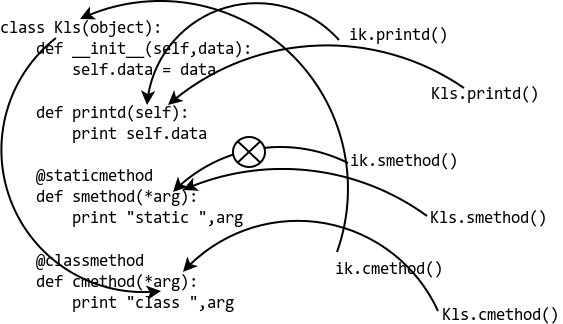# 零基础学Python:类(5)

• 方法是类内部定义函数，只不过这个函数的第一个参数是self。（可以认为方法是类属性，但不是实例属性）
• 必须将类实例化之后，才能通过实例调用该类的方法。调用的时候在方法后面要跟括号（括号中默认有self参数，但是不写出来。）

## 调用绑定方法

``````class Person(object):
def foo(self):
pass
``````

``````pp = Person()    #实例化
pp.foo()
``````

## 调用非绑定方法

《类(4)》中，介绍了一个函数super。为了描述方便，把代码复制过来：

``````#!/usr/bin/env python
# coding=utf-8

__metaclass__ = type

class Person:
def __init__(self):
self.height = 160

print "{} is about {}".format(name, self.height)

class Girl(Person):
def __init__(self):
super(Girl, self).__init__()
self.breast = 90

print "{} is a hot girl, she is about {}, and her breast is {}".format(name, self.height, self.breast)

if __name__ == "__main__":
cang = Girl()
``````

``````Person.__init__(self)
``````

## 静态方法和类方法

``````#!/usr/bin/env python
# coding=utf-8

__metaclass__ = type

class StaticMethod:
@staticmethod
def foo():
print "This is static method foo()."

class ClassMethod:
@classmethod
def bar(cls):
print "This is class method bar()."
print "bar() is part of class:", cls.__name__

if __name__ == "__main__":
static_foo = StaticMethod()    #实例化
static_foo.foo()               #实例调用静态方法
StaticMethod.foo()             #通过类来调用静态方法
print "********"
class_bar = ClassMethod()
class_bar.bar()
ClassMethod.bar()
``````

• `@staticmethod`表示下面的方法是静态方法
• `@classmethod`表示下面的方法是类方法

``````\$ python 21001.py
This is static method foo().
This is static method foo().
********
This is class method bar().
bar() is part of class: ClassMethod
This is class method bar().
bar() is part of class: ClassMethod
``````

### Class vs static methods in Python

staticmethod和classmethod均被作为装饰器，用作定义一个函数为”staticmethod”还是”classmethod”

### Simple, static and class methods

``````class Kls(object):
def __init__(self, data):
self.data = data

def printd(self):
print(self.data)

ik1 = Kls('arun')
ik2 = Kls('seema')

ik1.printd()
ik2.printd()
``````

``````arun
seema
````````````def get_no_of_instances(cls_obj):
return cls_obj.no_inst

class Kls(object):
no_inst = 0

def __init__(self):
Kls.no_inst = Kls.no_inst + 1

ik1 = Kls()
ik2 = Kls()

print(get_no_of_instances(Kls))
``````

``````2
``````

### The Python @classmethod

``````def iget_no_of_instance(ins_obj):
return ins_obj.__class__.no_inst

class Kls(object):
no_inst = 0

def __init__(self):
Kls.no_inst = Kls.no_inst + 1

ik1 = Kls()
ik2 = Kls()
print iget_no_of_instance(ik1)
``````

``````2
``````

``````class Kls(object):
no_inst = 0

def __init__(self):
Kls.no_inst = Kls.no_inst + 1

@classmethod
def get_no_of_instance(cls_obj):
return cls_obj.no_inst

ik1 = Kls()
ik2 = Kls()

print ik1.get_no_of_instance()
print Kls.get_no_of_instance()
``````

We get the following output:

``````2
2
``````

### The Python @staticmethod

``````IND = 'ON'

def checkind():
return (IND == 'ON')

class Kls(object):
def __init__(self,data):
self.data = data

def do_reset(self):
if checkind():
print('Reset done for:', self.data)

def set_db(self):
if checkind():
self.db = 'new db connection'

ik1 = Kls(12)
ik1.do_reset()
ik1.set_db()
``````

``````Reset done for: 12
``````

``````IND = 'ON'

class Kls(object):
def __init__(self, data):
self.data = data

@staticmethod
def checkind():
return (IND == 'ON')

def do_reset(self):
if self.checkind():
print('Reset done for:', self.data)

def set_db(self):
if self.checkind():
self.db = 'New db connection'
print('DB connection made for: ', self.data)

ik1 = Kls(12)
ik1.do_reset()
ik1.set_db()
``````

``````Reset done for: 12
``````

### How @staticmethod and @classmethod are different

``````class Kls(object):
def __init__(self, data):
self.data = data

def printd(self):
print(self.data)

@staticmethod
def smethod(*arg):
print('Static:', arg)

@classmethod
def cmethod(*arg):
print('Class:', arg)
``````

``````>>> ik = Kls(23)
>>> ik.printd()
23
>>> ik.smethod()
Static: ()
>>> ik.cmethod()
Class: (<class '__main__.Kls'>,)
>>> Kls.printd()
TypeError: unbound method printd() must be called with Kls instance as first argument (got nothing instead)
>>> Kls.smethod()
Static: ()
>>> Kls.cmethod()
Class: (<class '__main__.Kls'>,)
``````## 文档字符串

``````"""This is python lesson"""

def start_func(arg):
"""This is a function."""
pass

class MyClass:
"""Thi is my class."""
def my_method(self,arg):
"""This is my method."""
pass
``````